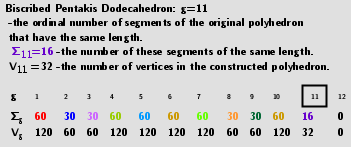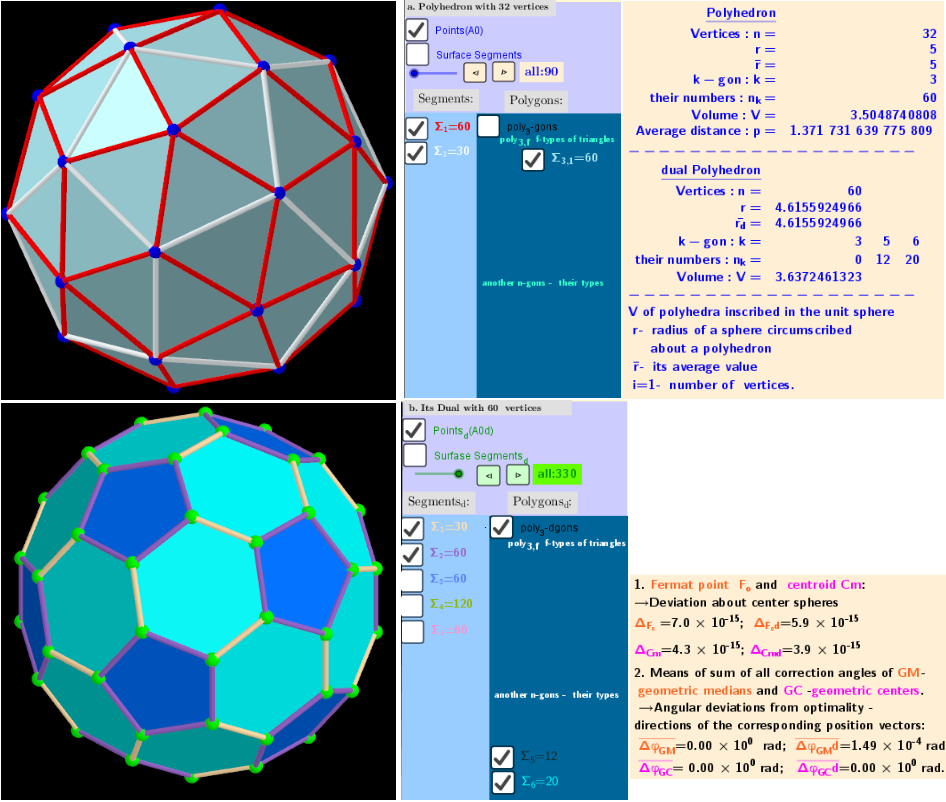# Biscribed Pentakis Dodecahedron (V=32) from Biscribed Pentakis Dodecahedron for the case of a trisection of its 11th-order segments

A polyhedron is constructed whose V=32 vertices are the points of the trisection of the segments the same length 11th-order (g=11) of the Biscribed Pentakis Dodecahedron. Geometric Constructions are in Applet: Series of polyhedra obtained by trisection (truncation) different segments of the original polyhedron, and the resulting polyhedra in Applet: Serie of polyhedra obtained by trisection (truncation) segments of the Biscribed Pentakis Dodecahedron.## 3. Properties of polyhedraBiscribed Pentakis Dodecahedron Vertices: 32 (12 + 20) Faces: 60 (isosceles triangles) Edges: 90 (60 short + 30 long) Dual Solid: Biscribed Truncated Icosahedron Vertices: 60 (60) Faces: 32 (12 regular pentagons + 20 ditrigons) Edges: 90 (30 short + 60 long)
The vertices of the last trisection of the segments of the original polyhedron are again its vertices!